Test: Modelling of Control Systems- 2

# Test: Modelling of Control Systems- 2

Test Description

## 15 Questions MCQ Test GATE Electrical Engineering (EE) 2023 Mock Test Series | Test: Modelling of Control Systems- 2

Test: Modelling of Control Systems- 2 for Electronics and Communication Engineering (ECE) 2023 is part of GATE Electrical Engineering (EE) 2023 Mock Test Series preparation. The Test: Modelling of Control Systems- 2 questions and answers have been prepared according to the Electronics and Communication Engineering (ECE) exam syllabus.The Test: Modelling of Control Systems- 2 MCQs are made for Electronics and Communication Engineering (ECE) 2023 Exam. Find important definitions, questions, notes, meanings, examples, exercises, MCQs and online tests for Test: Modelling of Control Systems- 2 below.
Solutions of Test: Modelling of Control Systems- 2 questions in English are available as part of our GATE Electrical Engineering (EE) 2023 Mock Test Series for Electronics and Communication Engineering (ECE) & Test: Modelling of Control Systems- 2 solutions in Hindi for GATE Electrical Engineering (EE) 2023 Mock Test Series course. Download more important topics, notes, lectures and mock test series for Electronics and Communication Engineering (ECE) Exam by signing up for free. Attempt Test: Modelling of Control Systems- 2 | 15 questions in 45 minutes | Mock test for Electronics and Communication Engineering (ECE) preparation | Free important questions MCQ to study GATE Electrical Engineering (EE) 2023 Mock Test Series for Electronics and Communication Engineering (ECE) Exam | Download free PDF with solutions
 1 Crore+ students have signed up on EduRev. Have you?
Test: Modelling of Control Systems- 2 - Question 1

### Match List - I (Control system components) with List - II (Principle of operation) and select the correct answer using the codes given below the lists: List - I A. Synchronous B. Potentiometer C. Tachometer List - lI 1. Mechanical displacement into electrical energy. 2. Angular position of a shaft into an electrical signal. 3. Electrical output proportional to speed. Codes: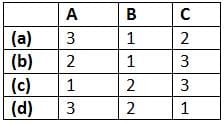Test: Modelling of Control Systems- 2 - Question 2

### An a.c. servomotor

Test: Modelling of Control Systems- 2 - Question 3

### Match List - I (Control System Components) with List - II (Applications) and select the correct answer using the codes given below the lists: List - I A. Stepper Motors B. Synchros C. Servomotors D. Potentiometer List - II 1. Radars, electromechanical actuators. 2. Displacement transducer 3. Printers, Watches 4. Detectors and encoders Codes: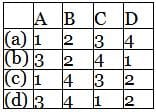Test: Modelling of Control Systems- 2 - Question 4

Match List - I (Types of friction) with List - II (Corresponding frictional force vs Velocity curve) and select the correct answer using the codes given below the lists: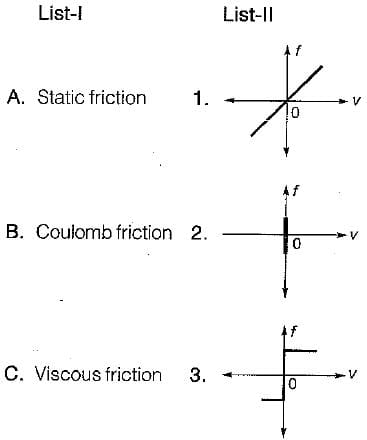Test: Modelling of Control Systems- 2 - Question 5

Assertion (A): D’Alembert’s principle states that “for any- body, the algebraic sum of externally applied forces and the forces resisting motion in any given direction is zero.
”Reason (R): D’Alembert principle is used in writing the equation of motion of mechanical systems.

Detailed Solution for Test: Modelling of Control Systems- 2 - Question 5

Both assertion and reason are true. However, the correct reason is that all the forces in the direction of reference direction are considered as positive and the forces opposite to the reference direction are taken as negative due to which the algebraic sum of forces is zero.

Test: Modelling of Control Systems- 2 - Question 6

Assertion (A): When two time constant elements are cascaded interactively, the overall transfer function of such an arrangement is the product of two individual transfer functions.

Detailed Solution for Test: Modelling of Control Systems- 2 - Question 6

When two time constant elements are cascaded non-interactively, then the overall transfer function is the product of two individual TFs.
Hence, assertion is false.

Test: Modelling of Control Systems- 2 - Question 7

Assertion (A): An a.c. servomotor used in control system applications must have high starting torque and low inertia.
Reason (R): A large value of rotor diameter and small axial length gives a higher value of starting torque and a low value of inertia.

Detailed Solution for Test: Modelling of Control Systems- 2 - Question 7

Reason is a false statement because small diameter and large axial length will give a lower value of inertia of motor.

Test: Modelling of Control Systems- 2 - Question 8

Assertion (A): When the rotor of the synchro is inclined along any one of the stator axis completely, then this position is called electrical zero position.
Reason (R): When the rotor of the synchro is inclined along any one of the stator axis completely, then maximum voltage will be induced in that winding and almost zero voltage will be induced in the other two windings.

Test: Modelling of Control Systems- 2 - Question 9

Assertion (A): Stepper motors can be used for speed control.
Reason (R): The motor speed is proportional to the rate of command pulses.

Detailed Solution for Test: Modelling of Control Systems- 2 - Question 9

Stepper motors can be used for speed control because the motor speed is proportional to the rate of command pulses.

Test: Modelling of Control Systems- 2 - Question 10

The stator of the synchros is made of

Test: Modelling of Control Systems- 2 - Question 11

A synchro is used to

Test: Modelling of Control Systems- 2 - Question 12

A synchro transmitter-receiver unit is a

Test: Modelling of Control Systems- 2 - Question 13

The transfer function of a tachometer is of the form

Test: Modelling of Control Systems- 2 - Question 14

In force-current analogy stiffness constant K is analogous to

Detailed Solution for Test: Modelling of Control Systems- 2 - Question 14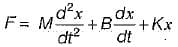and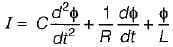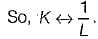Test: Modelling of Control Systems- 2 - Question 15

In liquid level systems, the units of capacitance of a tank is

Detailed Solution for Test: Modelling of Control Systems- 2 - Question 15

If q = flow; A = Area of cross-section and h = height of water in tank.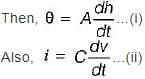On comparing equations (i) and (ii), we have C (Farad) = A = m2

## GATE Electrical Engineering (EE) 2023 Mock Test Series

22 docs|274 tests
Information about Test: Modelling of Control Systems- 2 Page
In this test you can find the Exam questions for Test: Modelling of Control Systems- 2 solved & explained in the simplest way possible. Besides giving Questions and answers for Test: Modelling of Control Systems- 2, EduRev gives you an ample number of Online tests for practice

## GATE Electrical Engineering (EE) 2023 Mock Test Series

22 docs|274 tests(Scan QR code)